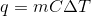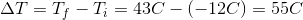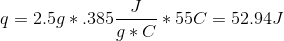# College Chemistry : Laws of Thermodynamics

## Example Questions

### Example Question #1 : Thermodynamics

The second law of thermodynamics states which of the following is true regarding an isolated system?

Two systems in thermal equilibrium with a third system are in thermal equilibrium with each other

The entropy approaches a constant value as the temperature of the system approaches zero Kelvin

The entropy can only decrease

Energy in the form of heat is always conserved

The entropy can only increase

The entropy can only increase

Explanation:

The entropy cannot decrease in an isolated system because the energy can only be degraded. Since the system is isolated, no higher-grade energy—or any energy at all—is being introduced into the system. As a result, the entropy cannot decrease. The other answer choices relate to the other laws of thermodynamics.

### Example Question #1 : Laws Of Thermodynamics

Which of the following statements is true of standard states?

Solute in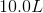of solution

Gas at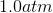pressure

Temperature at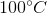Gas at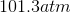Solute of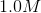concentration

Gas atpressure

Explanation:

Standard states are defined as a specific set of conditions, such as when a gas is atconcentration, and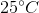.

Standard enthalpy of formation, the energy required for form 1 mole of a compound from its constituent elements, occurs when elements are in their standard states.

### Example Question #3 : Thermodynamics

How much heat is required to raise the temperature of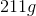of water from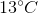to?  (Specific heat capacity of water is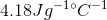)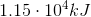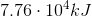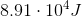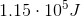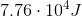Explanation: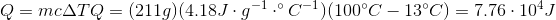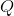is positive because heat flows into the system to raise the temperature of the water.

### Example Question #4 : Thermodynamics

How much heat is required to raise the temperature of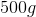of water from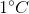to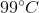? Specific heat capacity of water is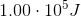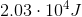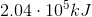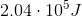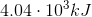Explanation: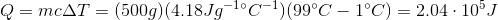### Example Question #5 : Thermodynamics

"In a natural thermodynamic process, the sum of the entropies of the interacting systems increases." Which law of thermodynamics does this statement refer to?

Zeroth law of thermodynamics

First law of thermodynamics

Second law of thermodynamics

Third law of thermodynamics

Second law of thermodynamics

Explanation:

There are four main laws of thermodynamics, which describe how temperature, energy, and entropy behave under various circumstances. The zeroth law of thermodynamics helps to define temperature; it states that if two systems are each in thermal equilibrium with a third system, they must be in thermal equilibrium with each other. The first law of thermodynamics negates the possibility of perpetual motion; it states that when energy passes into or out of a system, the system's internal energy changes in accord with the law of conservation of energy. The second law of thermodynamics also negates the possibility of perpetual motion; it states that in a natural thermodynamic process, the sum of the entropies of the interacting systems increases. Lastly, the third law of thermodynamics states that the entropy of a system approaches a constant value as the temperature nears absolute zero.

### Example Question #6 : Thermodynamics

Calculating heat

How much heat is absorbed by a copper penny as it warms from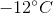to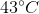assuming the penny is pure copper with a mass of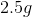?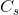of copper is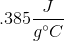.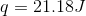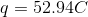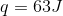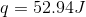Explanation:

Use the equation that relates heat, mass, specific heat, and change in temperature: